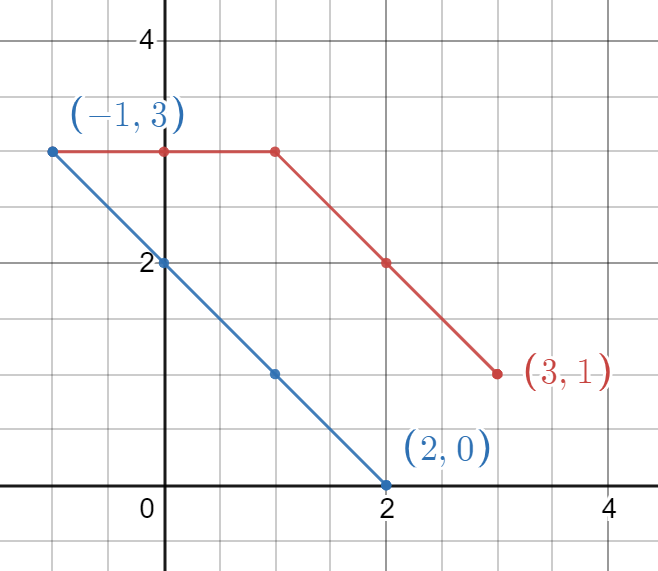New update is available. Click here to update.

# Minimum Time To Visit All Points

Last Updated: 25 Apr, 2021
Difficulty: Moderate

## PROBLEM STATEMENT

#### Ninja lives in a 2D plane where each location is represented with integer coordinates ‘[X, Y]’. You are given an array ‘POINTS’ containing ‘N’ such points. Ninja lives at the location ‘POINTS’ and wants to visit all the remaining ‘N-1’ points in the order given by the ‘POINTS’ array. Your task is to help ninja find the minimum time (in seconds) required to visit all the points in the given order (starting from ‘POINTS’).

##### For Example :
``````‘POINTS = [ [3, 1], [-1, 3], [2, 0] ]’, ‘N = 3’
````````````The path with minimum time is: ‘[3,1] -> [2,2] -> [1,3] - > [0,3] -> [-1,3] -> [0,2] -> [1,1] -> [2,0]’.

Time taken from [3,1] to [-1,3] = 4 seconds.
Time taken from [-1,3] to [2,0] = 3 seconds.

Total time = 7 seconds. Thus, you should return ‘7’ as the answer.
``````
##### Input Format :
``````The first line of input contains an integer ‘T’ which denotes the number of test cases. Then, the ‘T’ test cases follow.

The first line of each test case contains an integer ‘N’ denoting the number of points. Then, ‘N’ lines follow.

Each line contains two integers, ‘X’ and ‘Y’, representing an element of the array ‘POINTS’.
``````
##### Output Format :
``````For every test case, the minimum time to visit all the points in the given order.
``````
##### Note :
``````You do not need to print anything; it has already been taken care of. Just implement the function.
``````
##### Constraints :
``````1 <= T <= 100
1 <= N <= 1000

Each element of ‘POINTS’ contains exactly two integers ranging from [-10^5, 10^5].

Time limit: 1 second
``````